### Home > PC > Chapter 1 > Lesson 1.1.4 > Problem1-52

1-52.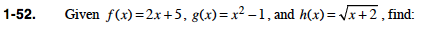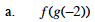Evaluate g(−2) first. Then substitute that value in the f function.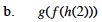$g(f(h(2)))= g(\sqrt{2 + 2})$

$=g(f(2))$

$=g(2(2) + 5)$

$= g(9)$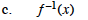1. Switch the x and y's
2. Solve for y.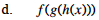Follow a similar process as shown in part (b).